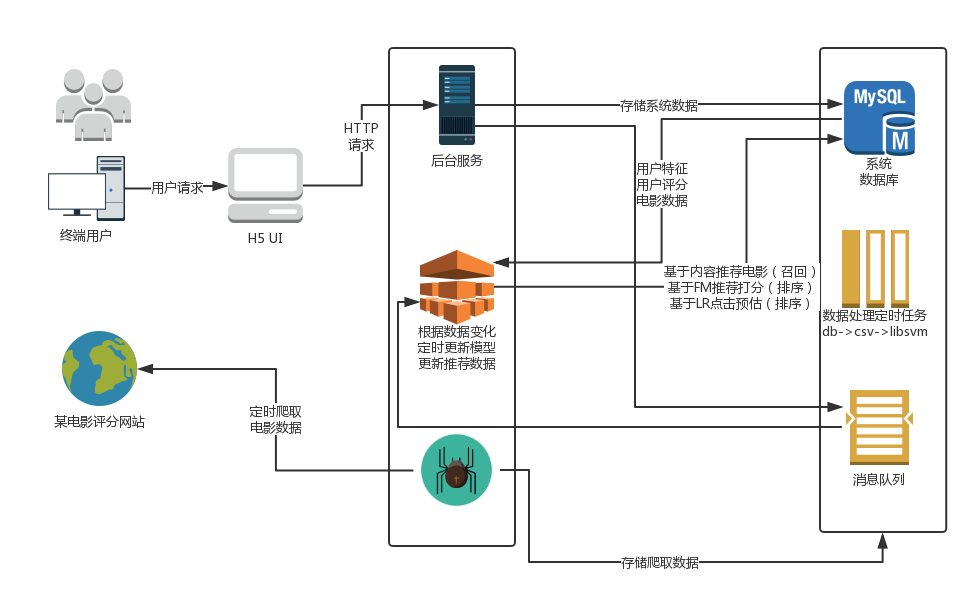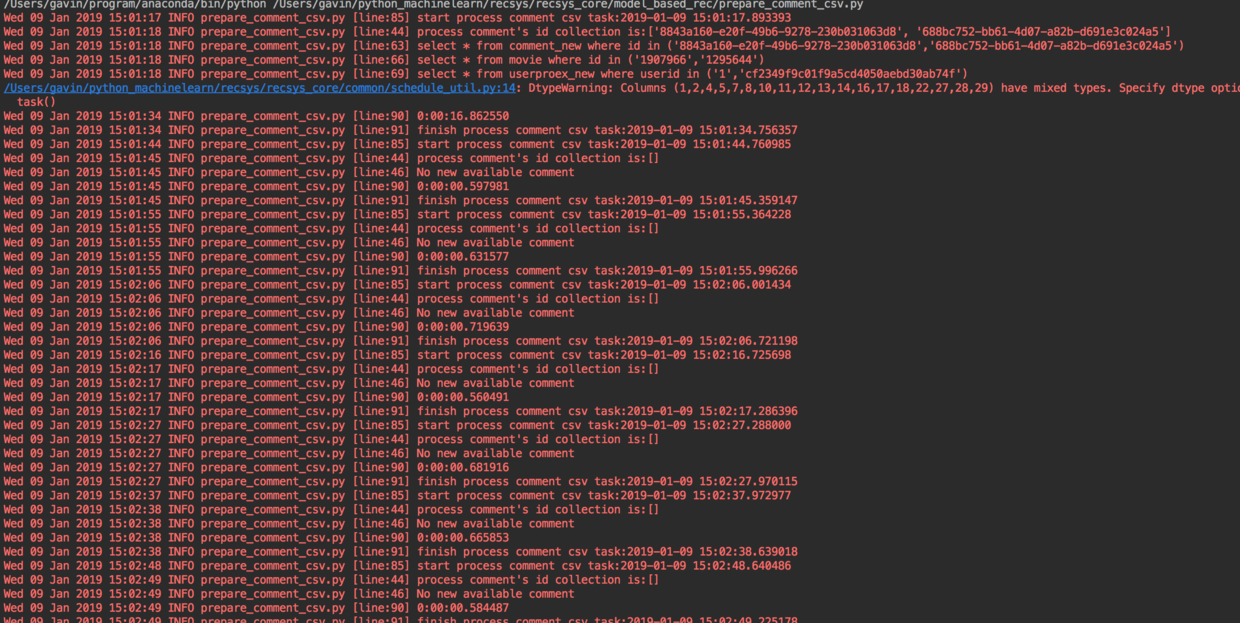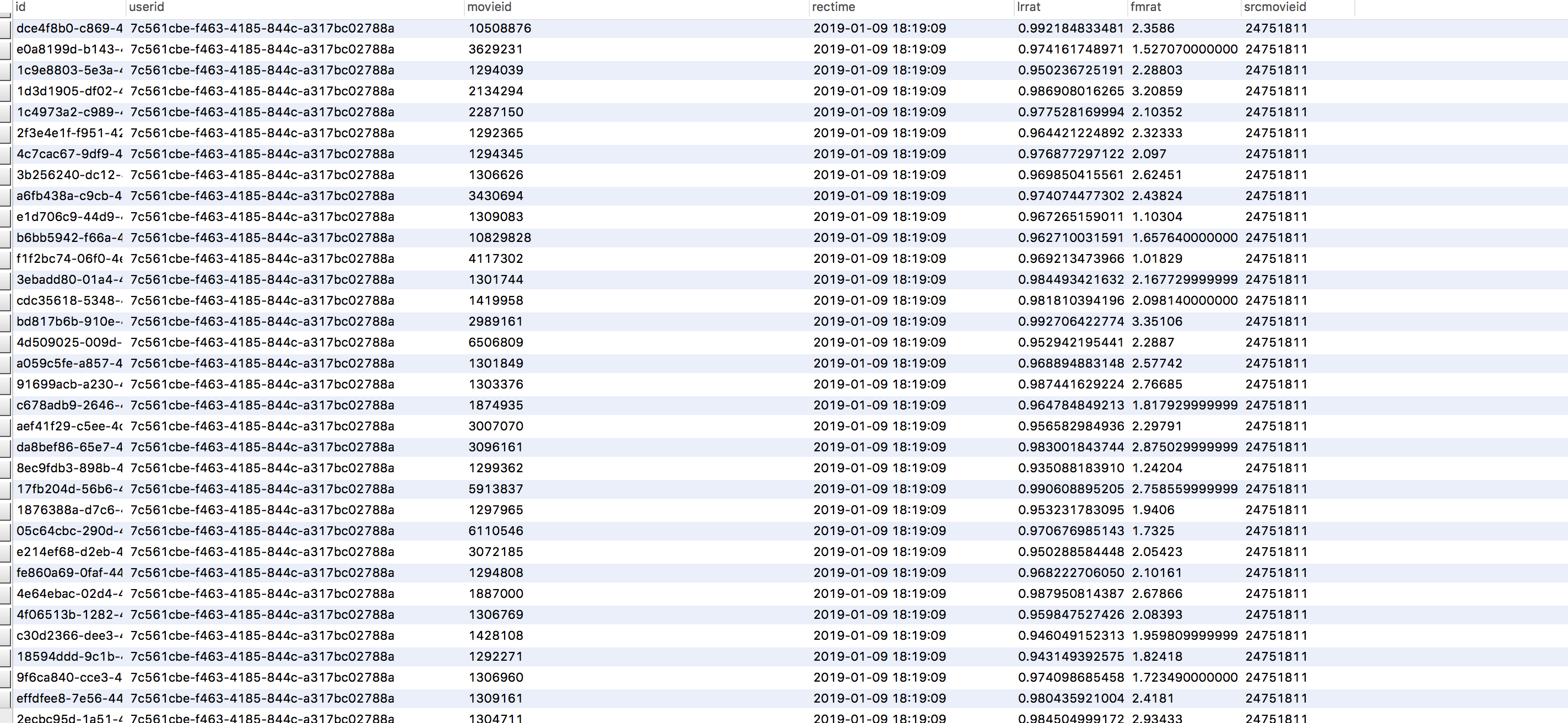# Python基于机器学习方法实现的电影推荐系统# 处理主函数，负责将多个冗余数据合并为一条电影数据，将地区，导演，主演，类型，特色等维度数据合并
def mainfunc():
try:
unable_list = []
with connection.cursor() as cursor:
sql='select id,name from movie'
cout=cursor.execute(sql)
print("数量： "+str(cout))

for row in cursor.fetchall():
#print(row)
movieinfo = df[df['电影名'] == row]
if movieinfo.shape == 0:
disable_movie(row)
print('disable movie ' + str(row))
else:
g = lambda x:movieinfo[x].iloc
types = movieinfo['类型'].tolist()
types = reduce(lambda x,y:x+'|'+y,list(set(types)))
traits = movieinfo['特色'].tolist()
traits = reduce(lambda x,y:x+'|'+y,list(set(traits)))
update_one_movie_info(type_=types, actors=g('主演'), region=g('地区'), director=g('导演'), trait=traits, rat=g('评分'), id_=row)

connection.commit()
finally:
connection.close()


'insert into userproex(userid, rmax, rmin, ravg, rcount, rsum, rmedian) values(\'%s\', %s, %s, %s, %s, %s, %s)' % (userid, rmax, rmin, ravg, rcount, rsum, rmedium)

'update userproex set rmax=%s, rmin=%s, ravg=%s, rmedian=%s, rcount=%s, rsum=%s where userid=\'%s\'' % (rmax, rmin, ravg, rmedium, rcount, rsum, userid)


def get_dim_dict(df, dim_name):
type_list = list(map(lambda x:x.split('|') ,df[dim_name]))
type_list = [x for l in type_list for x in l]
def reduce_func(x, y):
for i in x:
if i == y:
x.remove(i)
x.append(((i,i + 1)))
return x
x.append(y)
return x
l = filter(lambda x:x != None, map(lambda x:[(x, 1)], type_list))
type_zip = reduce(reduce_func, list(l))
type_dict = {}
for i in type_zip:
type_dict[i] = i
return type_dict


df_ = df.drop(['ADD_TIME', 'enable', 'rat', 'id', 'name'], axis=1)


movie_dict_list = []
for i in df_.index:
movie_dict = {}
#type
for s_type in df_.iloc[i]['type'].split('|'):
movie_dict[s_type] = 1
#actors
for s_actor in df_.iloc[i]['actors'].split('|'):
if actors_dict[s_actor] < 2:
movie_dict['other_actor'] = 1
else:
movie_dict[s_actor] = 1
#regios
movie_dict[df_.iloc[i]['region']] = 1
#director
for s_director in df_.iloc[i]['director'].split('|'):
if director_dict[s_director] < 2:
movie_dict['other_director'] = 1
else:
movie_dict[s_director] = 1
#trait
for s_trait in df_.iloc[i]['trait'].split('|'):
movie_dict[s_trait] = 1
movie_dict_list.append(movie_dict)


v = DictVectorizer()
X = v.fit_transform(movie_dict_list)


USERID	cf2349f9c01f9a5cd4050aebd30ab74f
movieid	10533913
type	剧情|奇幻|冒险|喜剧
actors	艾米·波勒|菲利丝·史密斯|理查德·坎德|比尔·哈德尔|刘易斯·布莱克
region	美国
director	彼特·道格特|罗纳尔多·德尔·卡门
trait	感人|经典|励志
rat	8.7
rmax	5
rmin	2
ravg	3.85714
rcount	7
rmedian	4
TIME_DIS	15


dump_svmlight_file(train_X_scaling, train_y_, train_file)


## 模块列表

• recsys_ui: 前端技术(html5+JavaScript+jquery+ajax)
• recsys_web: 后端技术(Java+SpringBoot+mysql)
• recsys_spider: 网络爬虫(python+BeautifulSoup)
• recsys_sql: 使用SQL数据处理
• recsys_model: pandas, libFM, sklearn. pandas数据分析和数据清洗，使用libFM,sklearn对模型初步搭建
• recsys_core: 使用pandas, libFM, sklearn完整的数据处理和模型构建、训练、预测、更新的程序
• recsys_etl：ETL 处理爬虫增量数据时使用kettle ETL便捷处理数据

# www.technologyx.cn

gavin 123
gavin2 123
wuenda 123## 设计思路#### 用简单地方式表述一下设计思路，

##### 5.推荐模块recsys_core会拉取用户的打分消息，并且并行的做以下操作：
a.增量的更新训练样本
b.快速（因服务器比较卡，目前设定了延时）对用户行为进行基于内容推荐的召回
c.训练样本更新模型
d.使用FM，LR模型对Item based所召回的数据进行精排
e.处理新用户注册消息，监听到用户注册消息后，对该用户的属性初始化(统计值)。
f.处理新电影添加消息，更新基于内容相似度而生成的相似度矩阵


## 模型相关的模块介绍

##### 增量的处理用户comment，即增量处理评分模块##### 把csv处理为libsvm数据##### 基于内容相似度推荐##### libFM预测

http://www.libfm.org/##### 逻辑回归预测posted @ 2019-06-23 18:05  GavinHacker  阅读(4882)  评论(2编辑  收藏